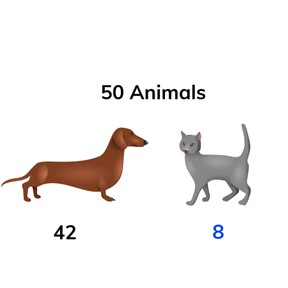Decomposing and composing to the tens place

# Decomposing and composing to the tens place

Decomposing and composing to the tens place

No account needed.8,000 schools use Gynzy92,000 teachers use Gynzy1,600,000 students use Gynzy

## General

Students practice decomposing random numbers to 90 by counting on to the next ten.

## Relevance

It is useful to be able to do this so you know how you can divide a number into two. An example- there are 50 animals in the shelter, of which 42 are dogs and the rest cats. If you decompose 50 you know that there are 8 cats.

## Introduction

Students practice counting on to the next ten. Select a student- they may choose a number from the circle and count on to the next ten. They then select the next student who gets to pick a number and count to the next ten. Repeat until all the numbers have been used.

## Development

Demonstrate step by step how to decompose 40 using the eggs. Which number has been given? Count that first. Then count on from 34 how many you still need and use that number to complete the number bond. That means you've decomposed 40 into 34 and 6. Discuss that composing is done when you count on without seeing the whole total. Students are asked to count in their heads the amount of eggs needed to reach the next ten. Explain in context that you can decompose 30 into 24 and 6. You count on from 24 to the next 10 by skip counting on the number line. You first show the single counts to reach the ten, and then show the jump of 6. Next, show the number bond chart for 30. Point out that the numbers in the left column keep going up by one and the numbers in the right column go down by one. Ask students if they can come up with other decompositions of 30. Repeat this practice while decomposing 60. Check if students can decompose without story context or visual support. The students are asked to count on in their heads.

Check that students have understood decomposing and composing to the nearest ten by asking the following questions:
- How can you easily decompose to a 10? (take the number that you are given, and count on to the nearest ten.)
- Why is it useful to be able to decompose numbers?

## Guided Practice

Students first practice composing to the nearest ten with an image. Then they practice decomposing to tens without an image, and finally completing number bond charts.

## Closing

Students practice composing to a given tens number. They then complete the number bond charts. Discuss why it is important to be able to decompose and how do do this easily to the nearest ten.

## Teaching Tip

Students who have difficulty decomposing can be supported by manipulatives (MAB). You can make the tens and ones tangible for the students as you compose to the nearest ten.

## Instruction materials

MAB- blocks

### The online teaching platform for interactive whiteboards and displays in schools

• Save time building lessons

• Manage the classroom more efficiently

• Increase student engagement